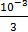# Two coils ‘P’ and ‘Q’ are separated by some distance.  When a current 3 A flows through the coil ‘P’, a magnetic flux ofWb passes through Q.  No current is passed through ‘Q’.  When no current passes through ‘P’ and a current of 2 A passes through ‘Q’, the flux through ‘P’ is (a) 6.6710−3 Wb (b) 6.6710−4 Wb (c) 3.6710−3 Wb (d) 3.6710−4  Wb

## Question ID - 51305 :- Two coils ‘P’ and ‘Q’ are separated by some distance.  When a current 3 A flows through the coil ‘P’, a magnetic flux ofWb passes through Q.  No current is passed through ‘Q’.  When no current passes through ‘P’ and a current of 2 A passes through ‘Q’, the flux through ‘P’ is (a) 6.6710−3 Wb (b) 6.6710−4 Wb (c) 3.6710−3 Wb (d) 3.6710−4  Wb

3537=Mi

⇒103=M(3)=M(2)

∴=QP==6.67Wb.

Next Question :A starts crossing the field diagonally. After walking half the distance, he turns right, walks some distance and turns left. Which direction is A facing now ?

(A) North-East                     (B) North-West

(C) North                              (D) South-East